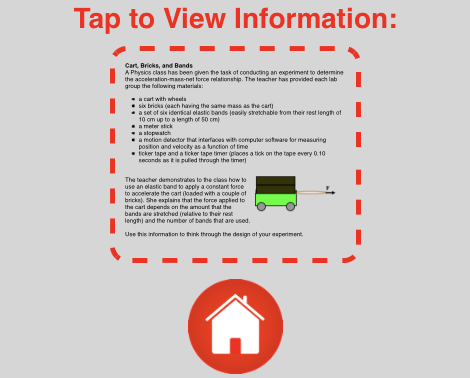## About the Cart, Bricks and BandsHighly Recommended
Like all our Science Reasoning Center activities, the completion of the Cart, Bricks, and Bands activity requires that a student use provided information about a phenomenon, experiment, or data presentation to answer questions. This information is accessible by tapping on the small thumbnails found on the bottom right of every question. However, it may be considerably easier to have a printed copy of this information or to display the information in a separate browser window. You can access this information from this page

The Standards
The Cart, Bricks, and Bands task describes a collection of equipment made available for investigating the effect of mass and of net force upon the acceleration of an object. This NGSS-inspired task consists of two activities. Each activity involves a different type of skill or understanding. Collectively, these two activities were designed to address the following NGSS performance expectation:

HS-PS2-1:
Analyze data to support the claim that Newton’s second law of motion describes the mathematical relationship among the net force on a macroscopic object, its mass, and its acceleration.

As a whole, the questions in this task address a wide collection of disciplinary core idea (DCI), crosscutting concepts (CCC), and science and engineering practices (SEP). There are 36 questions organized into 9 Question Groups and spread across the two activities. Each question is either a 2D or (preferrably) a 3D question. That is, the task of answering the question requires that the student utilize at least two of the three dimensions of the NGSS science standards - a DCI, a CCC, and/or an SEP.

The following DCI, SEPs, and CCCs are addressed at some point within Cart, Bricks, and Bands:

DCI:  PS2.A: Forces and Motion
Newton’s second law accurately predicts changes in the motion of macroscopic objects.

SEP 1.1:  Developing and Using Models
Ask questions that arise from careful observation of phenomena, or unexpected results, to clarify and/or seek additional information.

SEP 3.2: Planning and Carrying Out Investigations
Plan and conduct an investigation individually and collaboratively to produce data to serve as the basis for evidence, and in the design: decide on types, how much, and accuracy of data needed to produce reliable measurements and consider limitations on the precision of the data (e.g., number of trials, cost, risk, time), and refine the design accordingly.

SEP 4.1:  Analyzing and Interpreting Data

Analyze data using tools, technologies, and/or models (e.g., computational, mathematical) in order to make valid and reliable scientific claims or determine an optimal design solution.

SEP 4.3: Analyzing and Interpreting Data
Consider limitations of data analysis (e.g., measurement error, sample selection) when analyzing and interpreting data.

CCC 1.5:  Patterns
Mathematical representations are needed to identify some patterns.

CCC 3.2:  Scale, Proportion, and Quantity

Algebraic thinking is used to examine scientific data and predict the effect of a change in one variable on another (e.g., linear growth vs. exponential growth).

Here is our NGSS-based analysis of each individual activity of the Cart, Bricks, and Bands Science Reasoning task. The core ideas, crosscutting concepts, and science and engineering practices that we reference in our analysis are numbered for convenience. You can cross-reference the specific notations that we have used with the listings found on the following pages:

#### Part 1: Planning the Investigation

This activity consists of 16 forced-choice questions organized into four Question Groups. Students must evaluate a variety of experimental design questions.

NGSS Claim StatementPlan an investigation to produce data that serve as the basis for evidence regarding the rmathematical relationship between the mass of an object, the net force exerted on it, and the acceleration it experiences.

 Target DCI(s) Target SEP(s) Target CCC(s) Forces and Motion PS2.A Newton’s second law accurately predicts changes in the motion of macroscopic objects. Planning and Conducting an Investigation SEP 3.2 Plan and conduct an investigation individually and collaboratively to produce data to serve as the basis for evidence, and in the design: decide on types, how much, and accuracy of data needed to produce reliable measurements and consider limitations on the precision of the data (e.g., number of trials, cost, risk, time), and refine the design accordingly. Patterns CCC 1.5 Mathematical representations are needed to identify some patterns. Scale, Proportion, and Quantity CCC 3.2 Algebraic thinking is used to examine scientific data and predict the effect of a change in one variable on another (e.g., linear growth vs. exponential growth).

#### Part 2: Analyzing the Data

This activity consists of 20 forced-choice questions organized into five Question Groups. Students must make a variety of decisions regarding the analysis of data.

NGSS Claim Statement: Use a model to predict the effects of a change in one variable on another for objects that travel in elliptical orbits.

 Target DCI(s) Target SEP(s) Target CCC(s) Forces and Motion PS2.A Newton’s second law accurately predicts changes in the motion of macroscopic objects. Analyzing and Interpretting Data SEP 4.1 Analyze data using tools, technologies, and/or models (e.g., computational, mathematical) in order to make valid and reliable scientific claims or determine an optimal design solution. SEP 4.3 Consider limitations of data analysis (e.g., measurement error, sample selection) when analyzing and interpreting data. Patterns CCC 1.5 Mathematical representations are needed to identify some patterns.

Complementary and Similar Resources
The following resources at The Physics Classroom website complement the Cart, Bricks, and Bands Science Reasoning Activity. Teachers may find them useful for supporting students and/or as components of lesson plans and unit plans.

The Physics Classroom Tutorial, Newton's Laws: Newton's Second Law

Physics Video Tutorial, Newton's Laws: Newton's Second Law of Motion

Physics Interactives, Newton's Laws: Force

Physics Interactives, Newton's Laws: Falling Bodies 1D

Concept Builders, Newton's Laws of Motion: Newton's 2nd Law - Equations as a Guide to Thinking

Concept Builders, Newton's Laws of Motion: Net Force Ranking Tasks

Minds On Physics, Newton's Laws Module: Mission NL7, Newton's Second Law

The Calculator Pad, Newton's Laws: Problem Sets NL5 - NL10